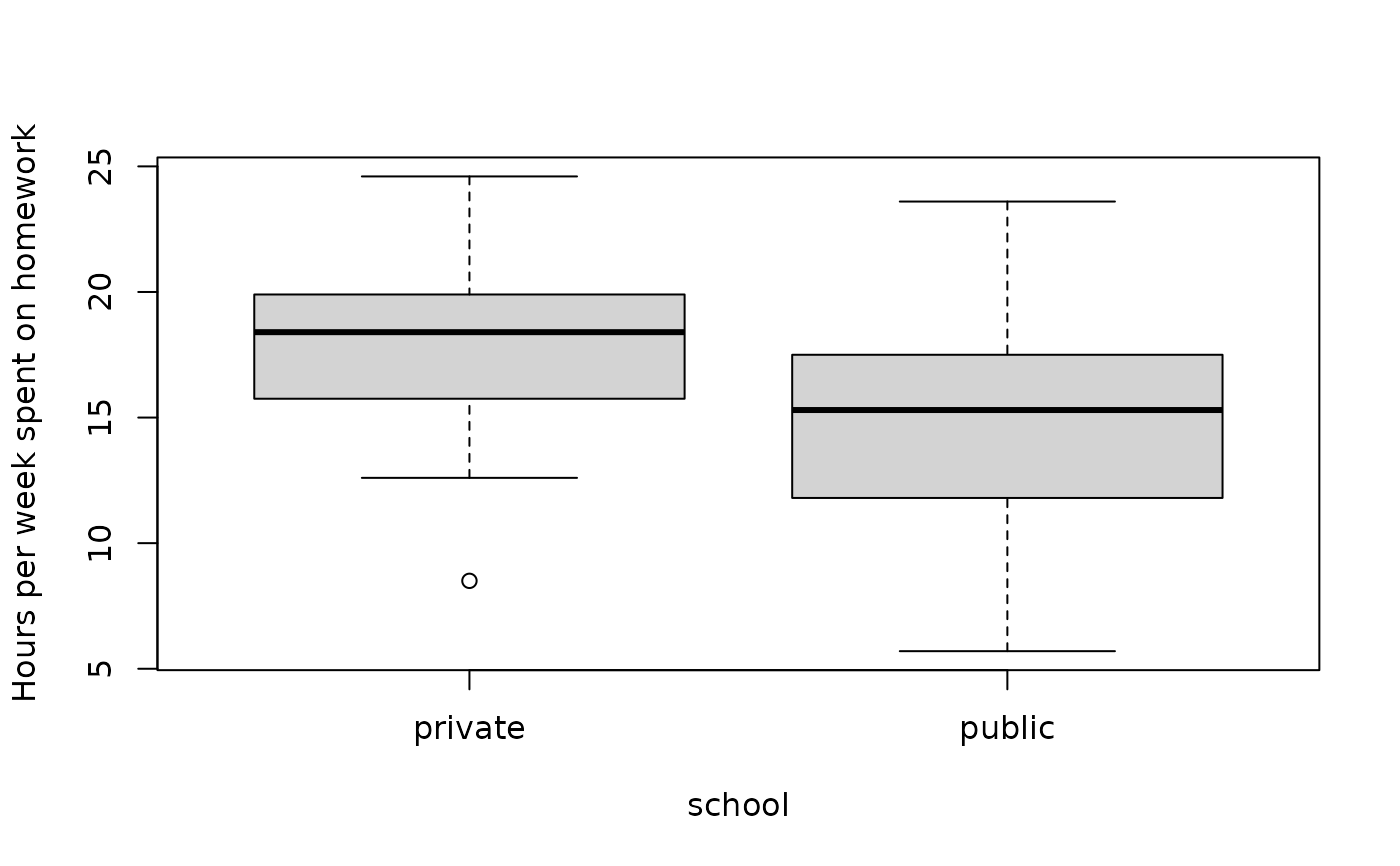Data for Exercise 7.78

Homework

## Format

A data frame with 30 observations on two variables

school

type of school either private or public

time

number of hours per week spent on homework

## Examples


boxplot(time ~ school, data = Homework,
ylab = "Hours per week spent on homework")#
t.test(time ~ school, data = Homework)
#>
#> 	Welch Two Sample t-test
#>
#> data:  time by school
#> t = 1.7134, df = 27.727, p-value = 0.09779
#> alternative hypothesis: true difference in means is not equal to 0
#> 95 percent confidence interval:
#>  -0.5345122  5.9878455
#> sample estimates:
#> mean in group private  mean in group public
#>              17.63333              14.90667
#>